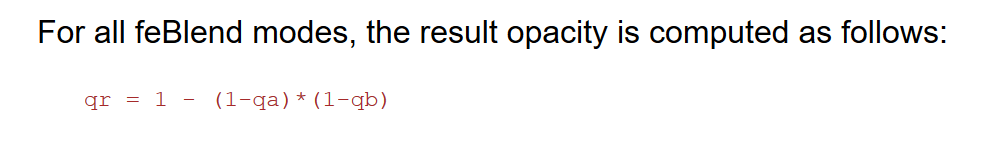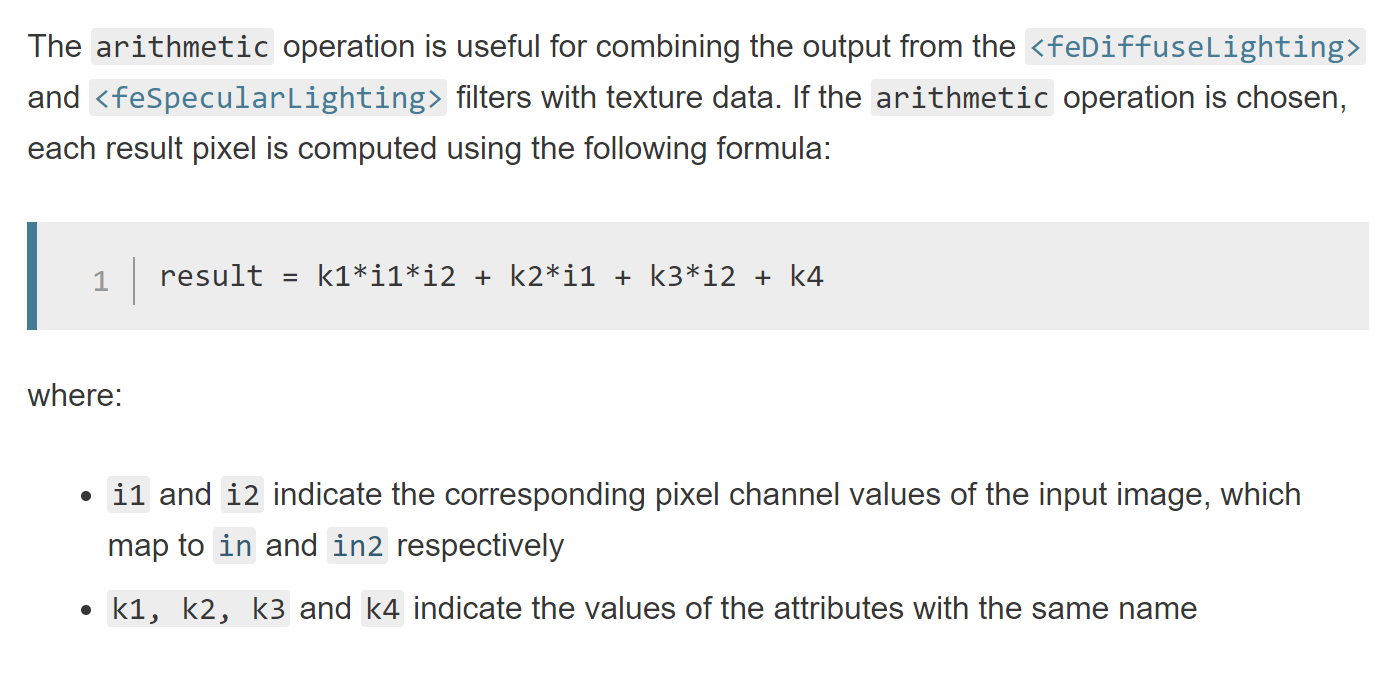## Using feColorMatrix to dynamically recolor icons (part 2, two-color icons)

Previously, I looked at how to use feColorMatrix to dynamically change the color of single-color icons. In this post, we’ll look at using feColorMatrix, feBlend, and feComposite to implement an algorithm that allow for dynamically changing the the colors of an icon with 2 colors.

#### The Algorithm

With a single color, we could think of the process as being a single step: applying the color transformation matrix with the desired R, G, B values. For two colors, there are multiple steps and matrices involved, so it’s worth having a high-level overview and conceptual understanding of the algorithm before delving into the details.

• The input icon will have 2 color, black and white; black areas will be changed to colorA and white areas will be changed to colorB
• Add colorA to the source image (black areas will take on the new color, white areas will remain white), the result is imageA
• Invert the source image, then add colorB to it (black areas will become white and remain white, white areas will become black and take on the new color), the result is imageB
• Combine imageA and imageB, such that the alpha component from the source image is preserved, output the resultNote that from the above, we see the key operations that are needed:

• Invert
• Combine

#### Another look at the color transformation matrix for applying a single-color

Note that the transformation matrix used previously for the single-color case, only preserves the alpha component from the input. The R, G, and B components are thrown away:It doesn’t matter what color the input pixel is, applying the transformation matrix will result in those Rsrc Gsrc Bsrc input values being multiplied by zero, before the new/output R, G, B values are added in.

While this is fine for the single-color case, for the two-color algorithm to work, the distinction between the black areas and the white areas need to be kept intact, so we have to work with the Rsrc Gsrc Bsrc values from the input vector and preserve the distinction.

#### Making Rsrc Gsrc Bsrc part of the transformation

Modifying the transformation matrix to allow Rsrc Gsrc Bsrc to be part of the calculations requires the first 3 diagonal elements of the matrix to be non-zero.

The simplest case of this is the identity matrix:Let’s look at a few matrices that define transformations needed for the algorithm.The invert matrix:The above can be combined into a single transformation matrix, the invert & add colorK matrix:#### Putting aside and referencing intermediate results

Note that the algorithm requires us to create 2 independent images (imageA and imageB) and then subsequently combine them.

This can be accomplished by utilizing the result attribute available on SVG filter primitive elements. By specifying a result identifier we are able to apply a transformation and put aside the resultant image.

#### Combining the intermediate results

Combining intermediate results/images can’t be done with feColorMatrix, it’s simply not an operation that can be constructed as a transformation. To handle this, SVG provides the feBlend element with a number of predefined operations available via the mode attribute. Based on the color transformations done to create imageA and imageB (mainly that the areas that are not of concern, the background, are set to white [1,1,1]), the multiply operation will work to combine the images (not perfectly, there’s one big problem with the alpha channel, but we’ll deal with that in a bit).```<feBlend color-interpolation-filters="linearRGB" in="imageA" in2="imageB" mode="multiply" result="output" /> ```

#### The alpha channel problem

While feBlend seem to accomplish what’s needed, for anything other than a white background you’ll notice a white-ist outline around elements in the image, as you can see below.The problem is that for what we’re trying to accomplish we just want to simply preserve the alpha channel, not blend it in any way. However, all feBlend operations will perform a blend of the alpha channel. From the SVG spec:So there’s no way we can really work with feBlend to get a solution for the alpha channel, but we do have an idea of what the solution is: copy the alpha channel from the source image.

#### Fixing the alpha channel

Fixing the alpha channel will involve 2 steps:

• For the image outputted by feBlend, get the alpha value to be 1 for every pixel
(the reason this step will become apparent once we look at how feComposite has to be used)
• Use feComposite to construct an image with the alpha values from the source image and the R,G,B values from the image outputted by feBlend

The first step is simple. We just need a slight modification to the 2 color transformation matrices used, such that one or both set the alpha channel to 1 for every pixel (note from the alpha blending equation, this will effectively set the alpha value to 1 for every pixel in the output). The modified matrices are shown below and it’s a good point to start showing some code.```<feColorMatrix in="SourceGraphic" type="matrix" result="imageA" values="1 0 0 0 0 0 1 0 0 0.68235294117 0 0 1 0 0.93725490196 0 0 0 0 1" /> ```

The full-alpha invert & add colorK matrix:```<feColorMatrix in="SourceGraphic" type="matrix" result="imageA" values="-1 0 0 0 1.0784313725 0 -1 0 0 1.7058823529 0 0 -1 0 1.2431372549 0 0 0 0 1" /> ```

For the next and final step, we need to take a look at feComposite.

The feComposite filter primitive allows for more fine-grained operations on pixels using the supported “arithmetic” operation:As this operation is done on every channel, including the alpha channel, we have way to control what happens to the alpha pixels in a composition of 2 images.

We’re going to make use of this by:

• Using an feColorMatrix, where the input in the source image, and transforming such that R,G,B is white [1,1,1] and the alpha remains unchanged
• Using feComposite to do a simple arithmetic multiply (k1=1, k2=0, k3=0, k4=0), between the image constructed above (where R,G,B is [1,1,1] and alpha is the alpha from the source image) and the output from feBlend (where the R,G,B = the values we want for the output and alpha = 1)

Effectively, the source alpha is multiplied by 1 (as the image produced from the feBlend operation has the alpha set to 1 for all pixels) and the R,G,B values from the feBlend output are multiplied by 1 (as the constructed image, in the first step above, sets R,G,B to 1 for every pixel).

```<!-- Get and use alpha from source image --> <feColorMatrix in="SourceGraphic" type="matrix" result="whiteAlpha" values="0 0 0 0 1 0 0 0 0 1 0 0 0 0 1 0 0 0 1 0" /> <feComposite in="whiteAlpha" in2="outputFullAlpha" operator="arithmetic" k1="1" k2="0" k3="0" k4="0" />```

#### Pulling everything together

We now have all the pieces for the filter and here’s what the code looks like:

```<svg style="width:0; height:0; margin:0; padding:0; border:none;"> <filter color-interpolation-filters="sRGB" id="colorTransformFilter"> <feColorMatrix in="SourceGraphic" type="matrix" result="imageA" values="1 0 0 0 0 0 1 0 0 0.68235294117 0 0 1 0 0.93725490196 0 0 0 0 1" /> <feColorMatrix in="SourceGraphic" type="matrix" result="imageB" values="-1 0 0 0 1.0784313725 0 -1 0 0 1.7058823529 0 0 -1 0 1.2431372549 0 0 0 0 1" /> <feBlend color-interpolation-filters="linearRGB" in="imageA" in2="imageB" mode="multiply" result="outputFullAlpha" /> <!-- Get and use alpha from source image --> <feColorMatrix in="SourceGraphic" type="matrix" result="whiteAlpha" values="0 0 0 0 1 0 0 0 0 1 0 0 0 0 1 0 0 0 1 0" /> <feComposite in="whiteAlpha" in2="outputFullAlpha" operator="arithmetic" k1="1" k2="0" k3="0" k4="0" /> </filter> </svg>```

The demo below uses the filter, along with a bit of Javascript to cycle and update the input colors (i.e. dynamically updating the values plugged into the first 2 feColorMatrix elements):

#### Limitations

We have the same limitations I mentioned in part 1:

• For icons applied as background-images, the CSS filter property isn’t ideal. CSS filter will effect not only the element it’s applied to, but all child elements as well
• As is the case with mixing the CSS filter property and the SVG filter element, effects governed by the CSS transition property won’t work

In addition, because of how the alpha channel in treated in regards to feBlend (setting all pixels to have alpha=1), you more than likely won’t get good results if the icon has different-colored adjoining or overlapping elements, as you won’t get a smooth transition at the edges/boundaries.